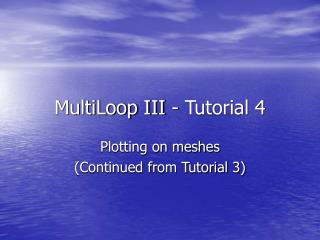# MultiLoop III - Tutorial 4 - PowerPoint PPT PresentationDownload PresentationMultiLoop III - Tutorial 4

MultiLoop III - Tutorial 4Download Presentation## MultiLoop III - Tutorial 4

- - - - - - - - - - - - - - - - - - - - - - - - - - - E N D - - - - - - - - - - - - - - - - - - - - - - - - - - -
##### Presentation Transcript

1. MultiLoop III - Tutorial 4 Plotting on meshes (Continued from Tutorial 3)

2. Step 1: Generate the plot data • Compute tutorials 1,2 and 3 • Edit the calculation data • Recalculate the response • Go

3. A view of the grid after tutorial 3

4. From the “Calculate” menu, select “Colours”

5. Save: Field data and stream potential coefficients will be saved to enable plotting later. Plot: Field data and stream potentials will be plotted as the calculations are being done. Apply to the primary field Apply to the inductive limit Apply to the resistive limit Apply to every nth time step Apply to the “gated stream potentials”. This option allows the stream potential that is responsible for the fields at each gate to be saved/viewed. The “Save Calculation Data” dialogue appears. If the colour table is not updated, the colours may not reflect the dynamic range of the stream potential currently being plotted. If it is updated, then the relative magnitudes of the stream potentials will be lost. During time stepping, not updating the colour table means that the late time stream potentials will be a uniform colour, because colour range will have been set by the first plot.

6. Select all save and plot options. Click “OK” when done.

7. The plotting coefficients must be computed before the plots can be made. From the “Calculate” menu, select “Response”

8. The calculation was done in Tutorial 1. The model has not changed, so the computed scattering data are still valid. Click “Yes” to recalculate and generate the plotting data.

9. The calculation restarts.

10. Because “Plot” was selected in the “Save Calculation Data” dialogue, the stream potentials are plotted as they are computed.

11. The first plots will be of the inductive and resistive limits.… Then plots for the time steps will be generated.

12. At late times, the current will diffuse away from the loop.

13. The final step is to compute and plot the gated stream potentials. When the calculation is done, a dialogue box will appear. Click “OK”.

14. Step 2: • Select “Plot”, “Colours” from the menu • The “Mesh Colouring Options” dialogue appears • Select the colouring option. • Go

15. To view the colour plots, select “Plot” and “Colours” from the main menu.

16. The “Mesh Colouring Options” dialogue appears

17. Click here to view the primary field normal to the mesh Click here to ensure that the colour table applies Click “Plot” to show the plot.

18. Click here to view the the inductive limit excitation Click here to ensure that the colour table applies Click “Plot” to show the plot.

19. A dialogue will appear with the available time steps (impulsive gates) available for plotting. Select the time to plot, click “OK” To plot a the stream potential at a time step, select “Time Step Excitation” Click the time select button, set at 0.00E+00 Click “Plot” when done

20. The selected stream potential is plotted and the time select button displays the time being plotted

21. The procedure for plotting the gated stream potential is similar. Click the “Gated Excitation” button. Then select the gate by clicking on the gate selector and select the gate from the dialogue.

22. The gated stream potential will be plotted, and the selected gate will be shown in the gate selector.